Ex 15.3

Chapter 15 Class 8 Introduction to Graphs
Serial order wise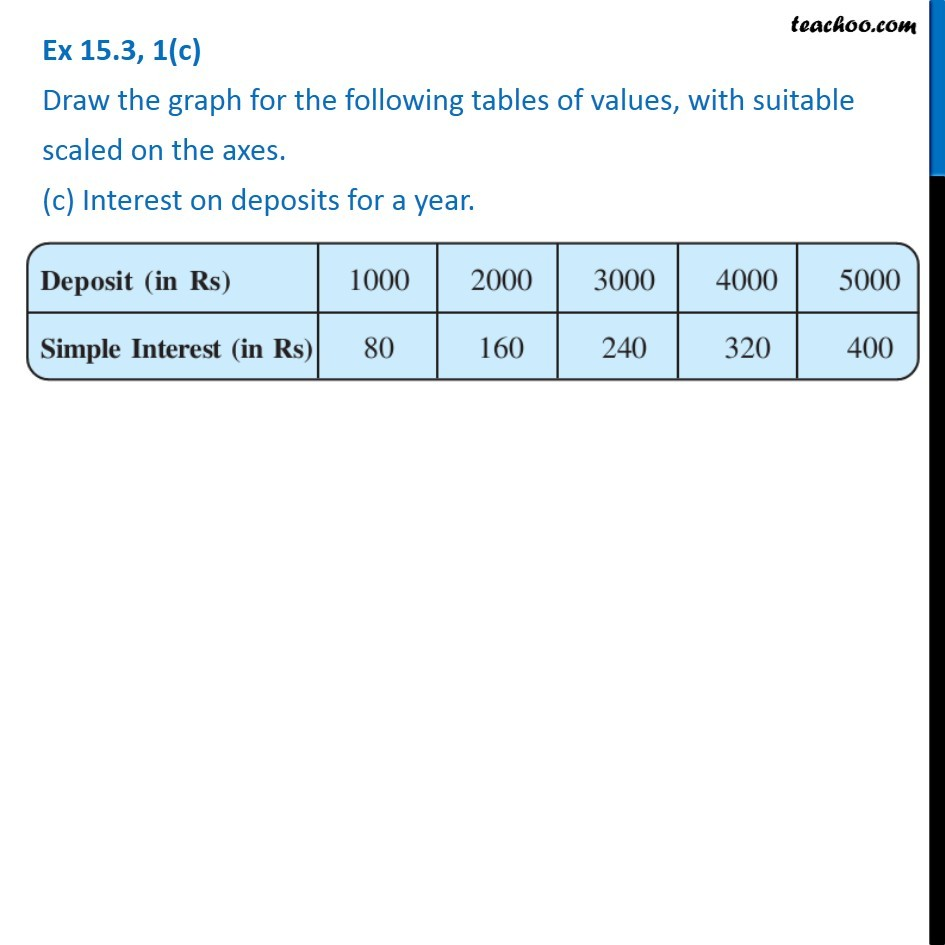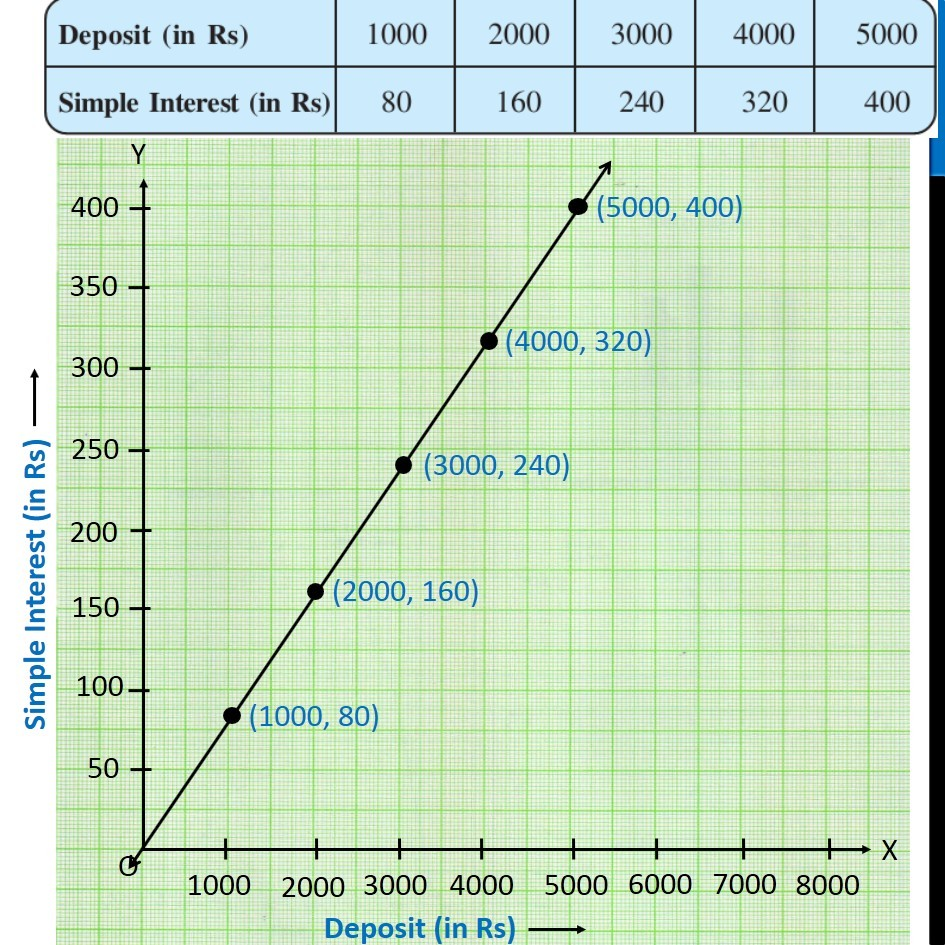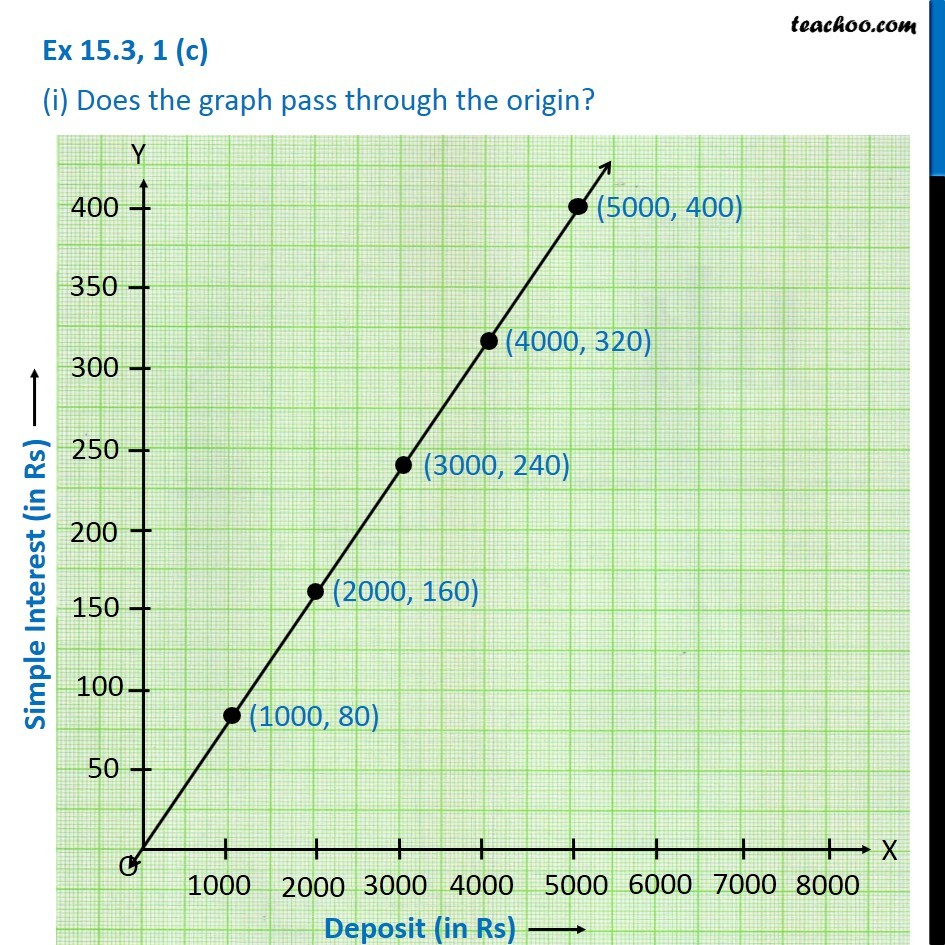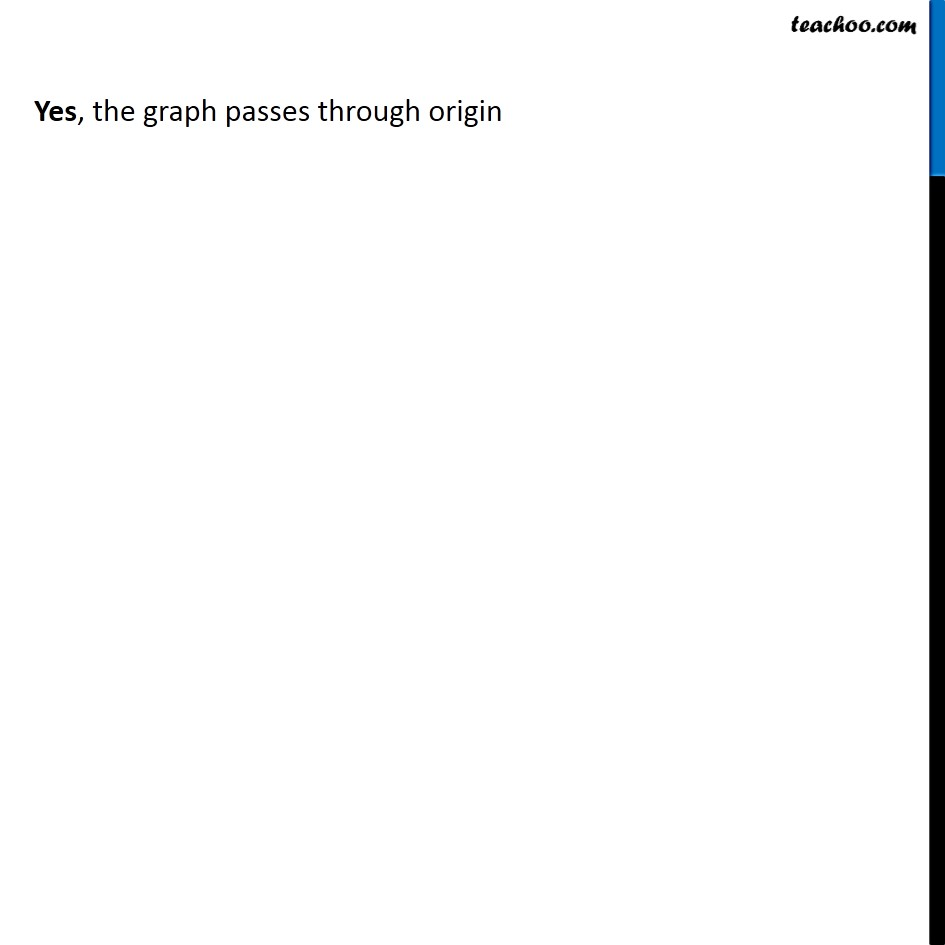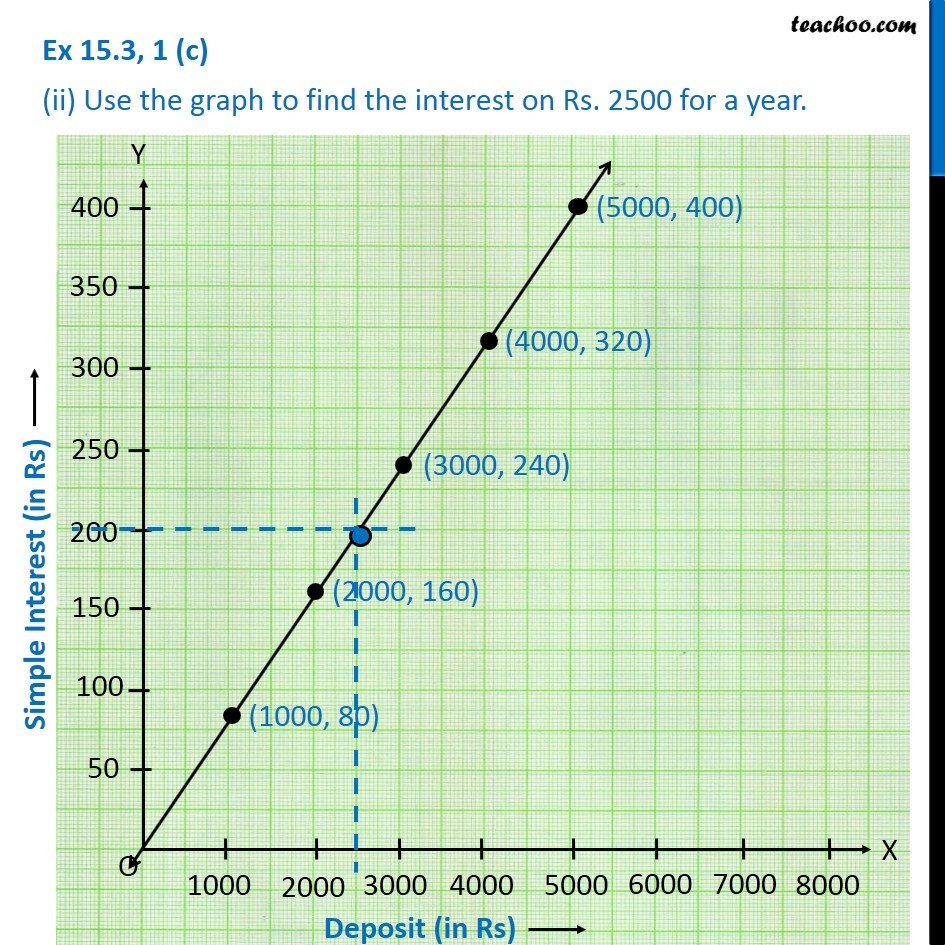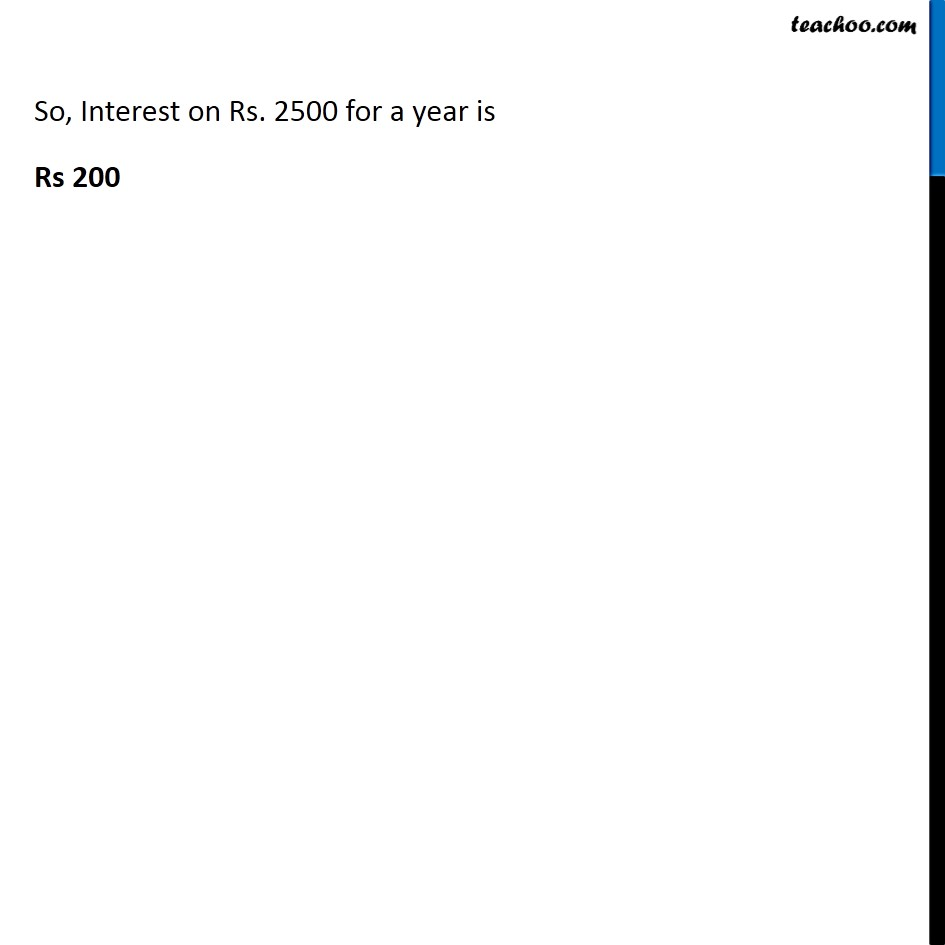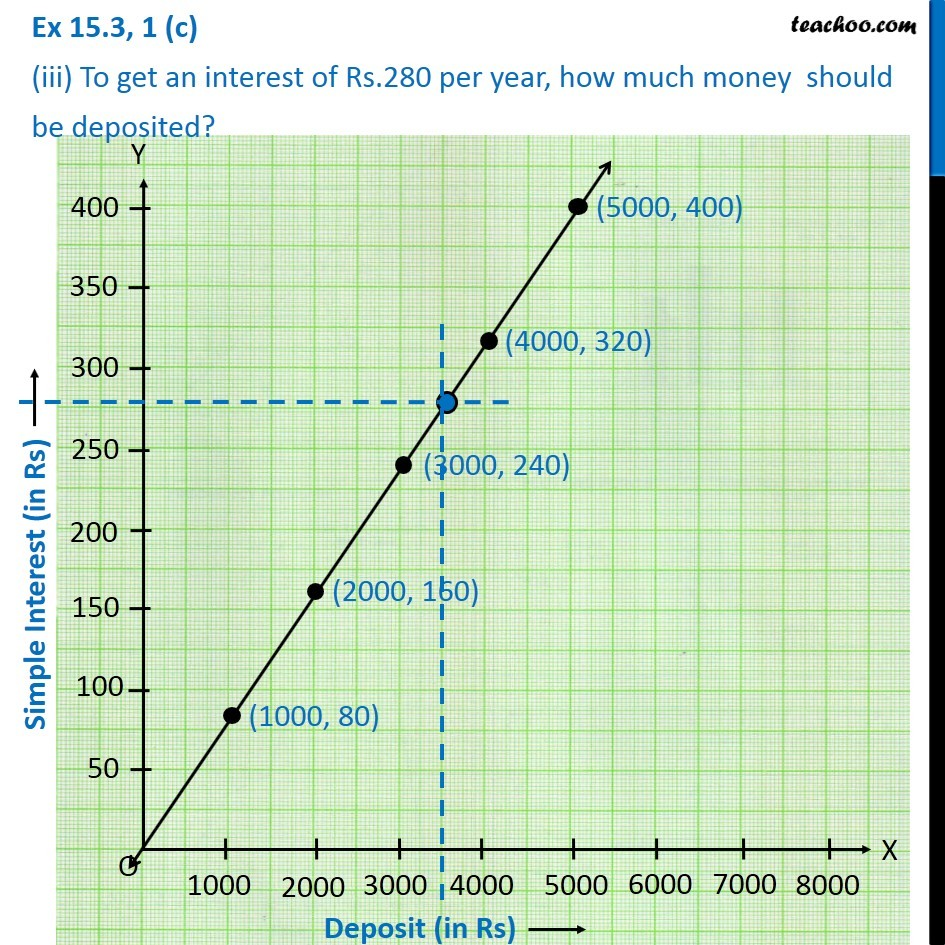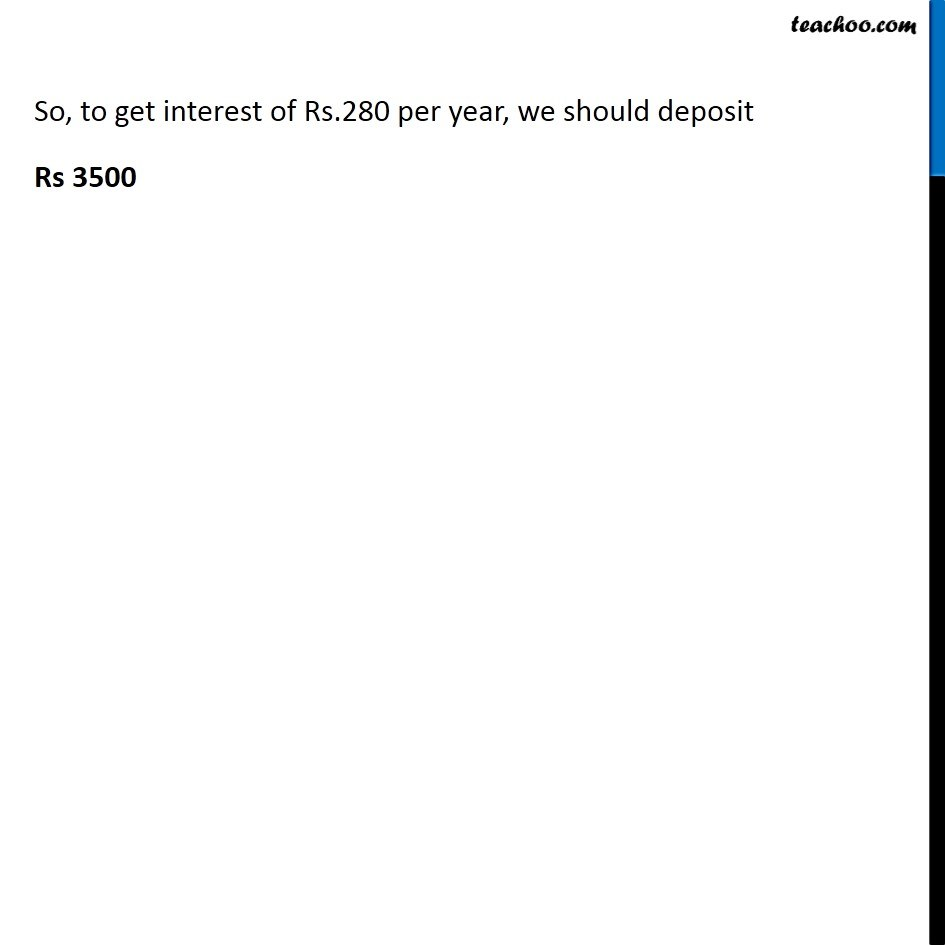Get live Maths 1-on-1 Classs - Class 6 to 12

### Transcript

Ex 15.3, 1(c) Draw the graph for the following tables of values, with suitable scaled on the axes. (c) Interest on deposits for a year. Deposit 1000 2000 3000 4000 5000 Simple Interest 80 160 240 320 400 Plotting Deposit in x-axis, from 0 to 8000 (with a gap of 1000) and Simple Interest (in Rs) from 0 to 400 (with a gap of 50) (i) Does the graph pass through the origin? Yes, the graph passes through origin (ii) Use the graph to find the interest on Rs. 2500 for a year. In x-axis, from 2500, draw a line parallel to y-axis, which cuts graph, From the point in the graph, Draw a line parallel to x-axis, where it cuts the y-axis is the interest So, Interest on Rs. 2500 for a year is Rs 200 (iii) To get an interest of Rs.280 per year, how much money should be deposited? In y-axis, from 280, draw a line parallel to x-axis, which cuts graph, From the point in the graph, Draw a line parallel to y-axis, where it cuts the x-axis is the deposit. Rs.280 per year, we should deposit Rs 3500